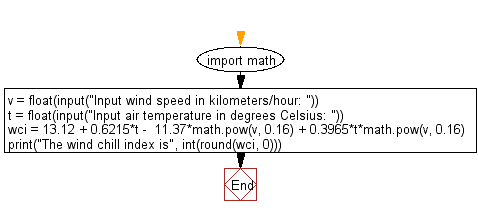﻿ Python Math: Calculate wind chill index - w3resource# Python Math: Calculate wind chill index

## Python Math: Exercise-29 with Solution

Write a Python program to calculate wind chill index.

Sample Solution:-

Python Code:

``````import math
v = float(input("Input wind speed in kilometers/hour: "))
t = float(input("Input air temperature in degrees Celsius: "))
wci = 13.12 + 0.6215*t -  11.37*math.pow(v, 0.16) + 0.3965*t*math.pow(v, 0.16)
print("The wind chill index is", int(round(wci, 0)))
```
```

Sample Output:

```Input wind speed in kilometers/hour: 120
Input air temperature in degrees Celsius: 35
The wind chill index is 40
```

Flowchart:## Visualize Python code execution:

The following tool visualize what the computer is doing step-by-step as it executes the said program:

Python Code Editor:

Have another way to solve this solution? Contribute your code (and comments) through Disqus.

What is the difficulty level of this exercise?

Test your Programming skills with w3resource's quiz.

﻿

```>>> students = [{'name': 'John', 'score': 98}, {'name': 'Mike', 'score': 94}, {'name': 'Jennifer', 'score': 99}]